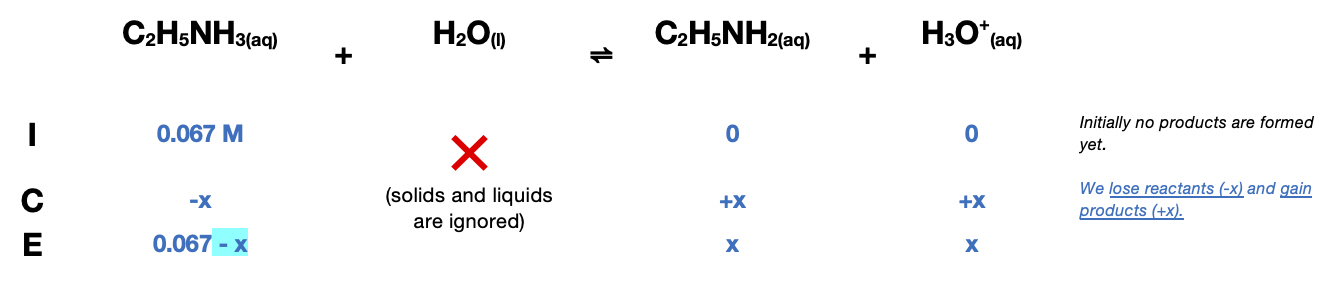# Problem: Calculate the pH at the halfway point and at the equivalence point for each of the following titrations.b. 100.0 mL of 0.10 M C2H5NH2 (Kb = 5.6 x 10 -4) titrated by 0.20 M HNO3

###### FREE Expert Solution

At halfway point,

Using the Henderson Hasselbalch equation:

$\mathbf{pH}\mathbf{=}{\mathbf{pK}}_{\mathbf{a}}\mathbf{=}\mathbf{-}\mathbf{log}\left(\frac{{10}^{-14}}{{K}_{b}}\right)\mathbf{=}$10.75

10 mmol

At the equivalence point, 10 mmol of HNO3 is added. The strong acid will convert the weak base completely to its conjugate acid:

50 mL

0.067 M$\overline{){{\mathbf{K}}}_{{\mathbf{a}}}{\mathbf{=}}\frac{\mathbf{products}}{\mathbf{reactants}}{\mathbf{=}}\frac{\left[{C}_{2}{H}_{5}{\mathrm{NH}}_{2}\right]\left[{H}_{3}{O}^{+}\right]}{{\mathbf{C}}_{\mathbf{2}}{\mathbf{H}}_{\mathbf{5}}{\mathbf{NH}}_{\mathbf{3}}}}$

Determine the value of Ka:

${\mathbf{K}}_{\mathbf{a}}\mathbf{=}\frac{{\mathbf{K}}_{\mathbf{w}}}{{\mathbf{K}}_{\mathbf{b}}}\mathbf{=}\frac{\mathbf{1}\mathbf{.}\mathbf{0}\mathbf{×}{\mathbf{10}}^{\mathbf{-}\mathbf{14}}}{\mathbf{5}\mathbf{.}\mathbf{6}\mathbf{×}{\mathbf{10}}^{\mathbf{-}\mathbf{4}}}\mathbf{=}$1.786×10-11

Substituting the equilibrium concentrations:

${\mathbf{1}}{\mathbf{.}}{\mathbf{78}}{\mathbf{×}}{{\mathbf{10}}}^{\mathbf{-}\mathbf{11}}{\mathbf{=}}\frac{\mathbf{\left(}\mathbf{x}\mathbf{\right)}\mathbf{\left(}\mathbf{x}\mathbf{\right)}}{\mathbf{0}\mathbf{.}\mathbf{067}\mathbf{-}\mathbf{x}}$

97% (291 ratings)###### Problem Details

Calculate the pH at the halfway point and at the equivalence point for each of the following titrations.

b. 100.0 mL of 0.10 M C2H5NH2 (Kb = 5.6 x 10 -4) titrated by 0.20 M HNO3